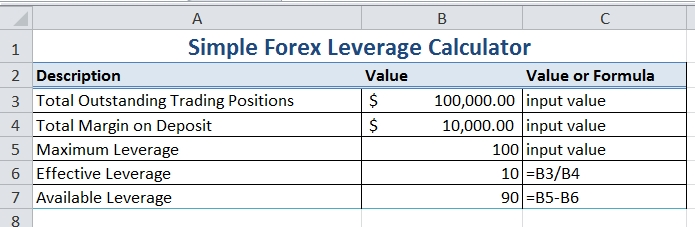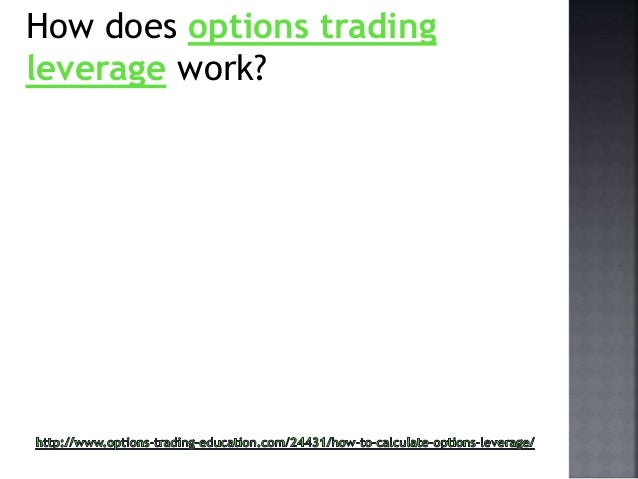# How To Trade Options With Leverage## How Leverage Works

Buying options contracts allows you to control a greater amount of the underlying security, such as stocks, than you could by actually trading the stocks themselves.

Put simply, if you had a certain amount of capital to invest then you can create the potential for far higher profits through buying options than you could through buying stocks.

This is essentially because the cost of options contracts is typically much lower than the cost of their underlying security, and yet you can benefit from price movements in the underlying security in the same way.

Let’s assume that you had \$1,000 to invest, and you wished to invest in Company X stock because you believed it was going to increase in price.

If Company X stock was trading at \$20, then you could purchase 50 shares in Company X with your \$1,000. If the stock went up in value, then you would be able to sell those shares for a profit. For example, if they went up by \$5 to \$25 then you could sell them at \$5 profit per share for a total profit of \$250 (not accounting for any commissions on your trades).

Now let’s assume you decided to invest in call options on Company X Stock, trading at \$2, with a strike price of \$20.

If the contract size was 100 you could buy five contracts at \$200 each: meaning you effectively have control over 500 shares in Company X (5 contracts, each covering 100 shares). This would mean that using your \$1,000 to buy options has given you control of 10 times as many shares as using your \$1,000 to directly buy shares at \$20 a time. With the price of Company X going up to \$25, you would make a lot more money through selling your options at a profit than the \$250 you would make in the example above.

That is essentially the principle of how leverage in options trading works, in very simple terms.

This should illustrate why it's possible to make significant profits without necessarily needing a lot of starting capital; which in turn is why so many investors choose to trade options. To truly understand leverage in greater detail, you need to understand how it's calculated, which we have explained below.

## Calculating Leverage

The examples given above of how investing \$1,000 directly in Company X stock compares to investing \$1,000 in call options on Company X stock, shows that buying options gave you control of 10 times as many shares.

## Options For Beginners: Understanding Leverage

A common misconception is that the leverage factor is then ten and you would therefore make ten times as much money.  However, that isn't actually the case.

The price of options contracts actually only moves a fraction of the amount that the price of the underlying security moves by.

Just because the underlying stock goes up by \$5 in the above example doesn’t necessarily mean that the corresponding contracts also go up by \$5. To understand how the price of options move in relation to the underlying security, you should be familiar with moneyness and how that affects one of the options Greeks: delta value.

The moneyness of options contracts relates to how much theoretical profit is currently built in to those contracts.

There are three states of moneyness: in the money, at the money, and out of the money.

In the money means the strike price is favorable compared to the price of the underlying security: i.e.

call options where the strike price is lower than the price of the underlying security are in the money options because there's effectively some built in profit.  At the money contracts are where the strike price is equal to the price of the underlying security, and out of the money contracts are where the strike price is unfavorable compared to the price of the underlying security.

The Delta value of an option is the ratio at which the price of the contract moves compared to the price of the underlying security.For example, the price of a contract with a delta value of 0.6 would move \$.60 for every \$1.00 move in the price of the underlying security.

In the money options contracts typically have a higher delta value than at the money contracts; they usually have a higher delta value than out of the money contracts. Once you understand all this, it's actually relatively straightforward to calculate leverage and determine how you want to use it when trading.The calculation for leverage is as follows:

(Delta Value of Option x Price of Underlying Security) / Price of Option

Let’s go back to the example we used above, where you were buying at the money call options on Company X stock at \$2 with a strike price of \$20 and Company X stock was trading at \$20. Assuming these contracts had a delta value of .5, the leverage would be calculated as follows:

Delta Value (.5) x Price of Underlying Security (\$20) = 10

Divided by the Price of Option (\$2) = 5

Therefore the leverage factor of these options contracts is 5, allowing you to make five times as much profit through buying options contracts as you would through buying the stock.Of course, this assumes that the stock does increase in price and the flip side to leverage is that it also multiplies potential losses too.

Basically, the higher the leverage factor, the greater the potential profits, but the greater the potential losses.

## Summary

The ability to use leverage to multiply potential profits is a huge advantage that trading options offers over trading many other financial instruments.

Best options for political emailing

However, it's important to recognize the increased risk that comes with using leverage.

Before you begin trading options you should understand how to calculate the leverage of taking any given position by using the delta value. You should also be aware of the role that moneyness plays in leverage and that out of the money contracts will have the highest leverage, followed by at the money options.  While in the money contracts have the lowest leverage.